## Overview

This guide introduces the local cubemap rendering techniques that are used to implement reflections in Unity version 5 and higher. Reflections are important in games because they make objects look realistic. Cubemaps offer several advantages compared to older rendering techniques. For example, cubemaps solve the image distortion problems that are associated with spherical mapping techniques.

At the end of this guide, you will have learned:

• How to implement reflections and refractions using local cubemaps
• How to combine static and dynamic reflections
• How to implement dynamic soft shadows using local cubemaps

## Implement reflections with a local cubemap

This section of the guide shows you how to combine reflections based on local cubemaps with other types of reflections. For example, reflections rendered at runtime in your own custom shader. Reflections that are based on a local cubemap are a useful technique for rendering reflections on mobile devices. Unity version 5 and higher implements reflections based on local cubemaps like `Reflection Probes`.

Graphics developers have always tried to find computationally cheap methods to implement reflections.

In 1999, it became possible to use cubemaps with hardware acceleration. Cubemaps solved the problems of image distortions, viewpoint dependency and, computational inefficiency which previously related to spherical mapping techniques.

Cubemapping uses the six faces of a cube as the map shape. The environment is projected onto each side of a cube and stored as six square textures or unfolded into six regions of a single texture. By rendering the scene from a given position with six different camera orientations with a 90° view frustum representing each a cube face, generating the cubemap. Source images are sampled directly. No distortion is introduced by resampling into an intermediate environment map.

The following image shows an unfolded cube:The following image shows infinite reflections:To implement reflections based on cubemaps, evaluate the reflected vector R and use it to fetch the texel from the cubemap. In this example, `_Cubemap`, uses the available texture lookup function `texCUBE()`:

`float4 color = texCUBE(_Cubemap, R);`

The normal `N` and view vector `D` are passed to the fragment shader from the vertex shader. The fragment shader fetches the texture color from the cubemap, as shown in the following code:

```float3 R = reflect(D, N);
float4 color = texCUBE(_Cubemap, R);
```

Implementing reflections based on cubemaps can only reproduce reflections correctly from a distant environment where the cubemap position is not relevant. This simple and effective technique is mainly used in outdoor lighting, for example, to add reflections of the sky.

The following image shows incorrect reflections: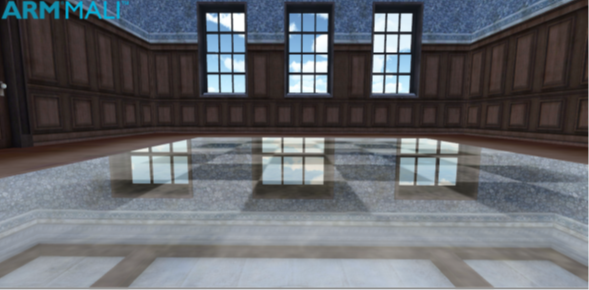If you use this infinite cubemaps in a local environment, it produces inaccurate reflections. The reflections are incorrect because in the following expression:

`float4 color = texCUBE(_Cubemap, R);`

There is no binding to the local geometry. For example, if you walk across a reflective floor looking at it from the same angle you always see the same reflection. The reflected vector is always the same and the expression always produces the same result. This is because the direction of the view vector does not change. In the real world, reflections depend on both viewing angle and viewing position.

#### Generate correct reflections with a local cubemap

A solution to the problem of incorrect reflections of local geometry involves binding to the local geometry in the procedure to calculate the reflection.

Note: This solution is described in GPU Gems: Programming Techniques, Tips, and Tricks for Real-time Graphics by Randima Fernando (Series Editor).

A bounding sphere is used as a proxy volume to delimit the scene that needs to be reflected. Instead of using the reflected vector R to fetch the texture from the cubemap a new vector R’ is used. To build the new vector, find the intersection point P in the bounding sphere of the ray, from the local point V in the direction of the reflected vector R. Create a new vector R’ from the center of the cubemap C, where the cubemap was generated, to the intersection point P.

The following image shows a local correction using a bounding sphere:Use this vector to fetch the texture from the cubemap:

```float3 R = reflect(D, N);
Find intersection point P
Find vector R’ = CP
float4 col = texCUBE(_Cubemap, R’);
```

This approach produces good results in the surfaces of objects with a near spherical shape but deforms reflections in plane reflective surfaces. Another problem with this method is that the algorithm that is used to calculate the intersection point with the bounding sphere involves solving a second-degree equation. This solution is relatively expensive.

In 2010 a developer proposed a better solution in a forum at Gamedev. The new approach replaces the previous bounding sphere with a box. This replacment solves the deformations and complexity problems of the previous method.

The following image shows a local correction using a bounding box:In 2012 Sebastien Lagarde used this approach to simulate more complex ambient specular lighting. This is done by using several cubemaps and an algorithm to evaluate the contribution of each cubemap and efficiently blend on the GPU.

The following table shows the differences between infinite and local cubemaps:

 Infinite Cubemaps Local Cubemaps Mainly used outdoors to represent the lighting from a distant environment Cubemap position is not relevant. Represents the lighting from a finite local environment Cubemap position is relevant. The lighting from local cubemaps is only correct at the location where the cubemap was created. Local correction must be applied to adapt the intrinsic infinite nature of cubemaps to local environment.

The following image is from the same scene as the one shown in the introductory paragraph. However, this version of the scene contains the correct reflections generated with local cubemaps: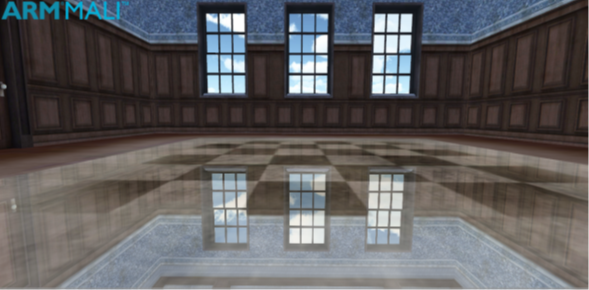This section shows shader implementation of reflections using local cubemaps in Unity.

The vertex shader calculates three magnitudes that are passed to the fragment shader as interpolated values:

• The vertex position
• The view direction
• The normal

These values are in world coordinates.

The following code shows a shader implementation of reflections using local cubemaps, for Unity:

```vertexOutput vert(vertexInput input)
{
vertexOutput output;
output.tex = input.texcoord;
// Transform vertex coordinates from local to world.
float4 vertexWorld = mul(_Object2World, input.vertex);
// Transform normal to world coordinates.
float4 normalWorld = mul(float4(input.normal,0.0), _World2Object);
// Final vertex output position. output.pos = mul(UNITY_MATRIX_MVP, input.vertex);
// ----------- Local correction ------------
output.vertexInWorld = vertexWorld.xyz;
output.viewDirInWorld = vertexWorld.xyz - _WorldSpaceCameraPos;
output.normalInWorld = normalWorld.xyz;
return output;
}
```

The intersection point in the volume box and the reflected vector are computed in the fragment shader. Build a new local corrected reflection vector and use it to fetch the reflection texture from the local cubemap. Then combine the texture and reflection to produce the output color:

```float4 frag(vertexOutput input) : COLOR
{
float4 reflColor = float4(1, 1, 0, 0);
// Find reflected vector in WS.
float3 viewDirWS = normalize(input.viewDirInWorld);
float3 normalWS = normalize(input.normalInWorld);
float3 reflDirWS = reflect(viewDirWS, normalWS);
// Working in World Coordinate System.
float3 localPosWS = input.vertexInWorld;
float3 intersectMaxPointPlanes = (_BBoxMax - localPosWS) / reflDirWS;
float3 intersectMinPointPlanes = (_BBoxMin - localPosWS) / reflDirWS;
// Looking only for intersections in the forward direction of the ray.
float3 largestParams = max(intersectMaxPointPlanes, intersectMinPointPlanes);
// Smallest value of the ray parameters gives us the intersection.
float distToIntersect = min(min(largestParams.x, largestParams.y), largestParams.z);
// Find the position of the intersection point.
float3 intersectPositionWS = localPosWS + reflDirWS * distToIntersect;
// Get local corrected reflection vector.
float3 localCorrReflDirWS = intersectPositionWS - _EnviCubeMapPos;
// Lookup the environment reflection texture with the right vector.
reflColor = texCUBE(_Cube, localCorrReflDirWS);
// Lookup the texture color.
float4 texColor = tex2D(_MainTex, input.tex);
return _AmbientColor + texColor * _ReflAmount * reflColor;
}
```

In the preceding fragment shader code, the magnitudes `_BBoxMax` and `_BBoxMin` are the maximum and minimum points of the bounding volume. The variable `_EnviCubeMapPos` is the position where the cubemap was created. Pass these values to the shader from the following script:

```[ExecuteInEditMode]
public class InfoToReflMaterial : MonoBehaviour
{
// The proxy volume used for local reflection calculations.
public GameObject boundingBox;
void Start()
{
Vector3 bboxLength = boundingBox.transform.localScale;
Vector3 centerBBox = boundingBox.transform.position;
// Min and max BBox points in world coordinates
Vector3 BMin = centerBBox - bboxLength/2;
Vector3 BMax = centerBBox + bboxLength/2;
// Pass the values to the material.
gameObject.renderer.sharedMaterial.SetVector("_BBoxMin", BMin);
gameObject.renderer.sharedMaterial.SetVector("_BBoxMax", BMax);
gameObject.renderer.sharedMaterial.SetVector("_EnviCubeMapPos", centerBBox);
}
}
```

Pass the values for `_AmbientColor`, `_ReflAmount`, the main texture, and cubemap texture to the shader as uniforms from the properties block:

```Shader "Custom/ctReflLocalCubemap"
{
Properties
{
_MainTex ("Base (RGB)", 2D) = "white" { }
_Cube("Reflection Map", Cube) = "" {}
_AmbientColor("Ambient Color", Color) = (1, 1, 1, 1)
_ReflAmount("Reflection Amount", Float) = 0.5
}
{
Pass
{
CGPROGRAM
#pragma glsl
#pragma vertex vert
#pragma fragment frag
#include "UnityCG.cginc"
// User-specified uniforms
uniform sampler2D _MainTex;
uniform samplerCUBE _Cube;
uniform float4 _AmbientColor;
uniform float _ReflAmount;
uniform float _ToggleLocalCorrection;
// ----Passed from script InfoRoReflmaterial.cs --------
uniform float3 _BBoxMin;
uniform float3 _BBoxMax;
uniform float3 _EnviCubeMapPos;
struct vertexInput
{
float4 vertex : POSITION;
float3 normal : NORMAL;
float4 texcoord : TEXCOORD0;
};
struct vertexOutput
{
float4 pos : SV_POSITION;
float4 tex : TEXCOORD0;
float3 vertexInWorld : TEXCOORD1;
float3 viewDirInWorld : TEXCOORD2;
float3 normalInWorld : TEXCOORD3;
};
ENDCG
}
}
}
```

The algorithm that is used to calculate the intersection point in the bounding volume is based on the use of the parametric representation of the reflected ray from the local position or fragment. For a description of the ray-box intersection algorithm, see Ray-box intersection algorithm

#### Filtering cubemaps

This section shows you to filter cubemaps after you have implemented reflections using local cubemaps using the CubeMapGen tool.

One of the advantages of implementing reflections using local cubemaps is the fact that the cubemap is static. This means that the cubemap is generated during development, rather than at runtime. Generating the cubemap during development provides an opportunity to apply any filtering to the cubemap images to achieve an effect.

To export cubemap images from Unity to CubeMapGen, you must save each cubemap image separately. It is best to use Legacy Cubemaps in Unity for this procedure, to make it easy to transfer individual images between the two tools.  For the source code of a tool that saves the images, see Source code to generate cubemaps. This tool can create a cubemap and can optionally save each cubemap image separately.

Note: CubeMapGen is a legacy tool by AMD that applies filtering to cubemaps.

You must place the script for this tool in a folder called Editor in the Asset directory.

To use the cubemap editor tool:

1. Create the cubemap.
2. Launch the Bake CubeMap tool from the GameObject menu.
3. Provide the cubemap and the camera render position.
4. Optionally save individual images if you plan to apply filtering to the cubemap.

The following screenshot shows the Bake CubeMap tool interface: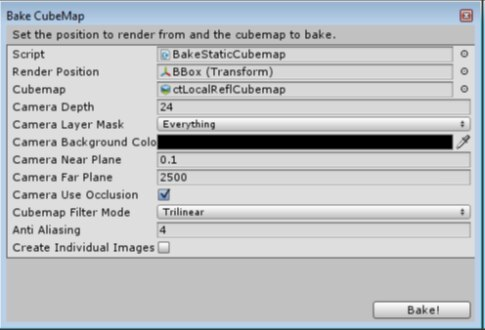You can load each of the images for the cubemap separately with CubeMapGen.

To load a face, select one from the Select Cube Face drop-down menu and then click Load Cubemap Face. When all faces have been loaded, rotate the cubemap and check that it is correct.

CubeMapGen has several filtering options in the Filter Type drop down menu. Select the filter settings that you require and click Filter cubemap to apply the filter. The filtering can take several minutes depending on the size of the cubemap.

Note: There is no undo option, so save the cubemap as a single image before applying any filtering. If the result of the filtering is not what you expect it to be, reload the cubemap and adjust the parameters.

Use the following procedure for importing cubemap images into CubeMapGen:

1. Make sure you have saved individual images by checking the Create Individual Images box when you baked the cubemap in Unity
2. Launch the CubeMapGen tool and load cubemap images following the relations that are shown in the following table:
 AMD CubeMapGen Unity X+ -X X- +X Y+ +Y Y- -Y Z+ +Z Z- -Z
3. Save the cubemap as a single dds or cube cross image.
Note: There is no undo, therefore save the cubemap as a single image before filtering, enabling you to reload the cubemap if you are experimenting with filters.
4. Apply filters to the cubemap as required until the results are satisfactory.
5. Save the cubemap as individual images.

The following screenshot shows the input image in CubeMapGen after loading the six cubemap images: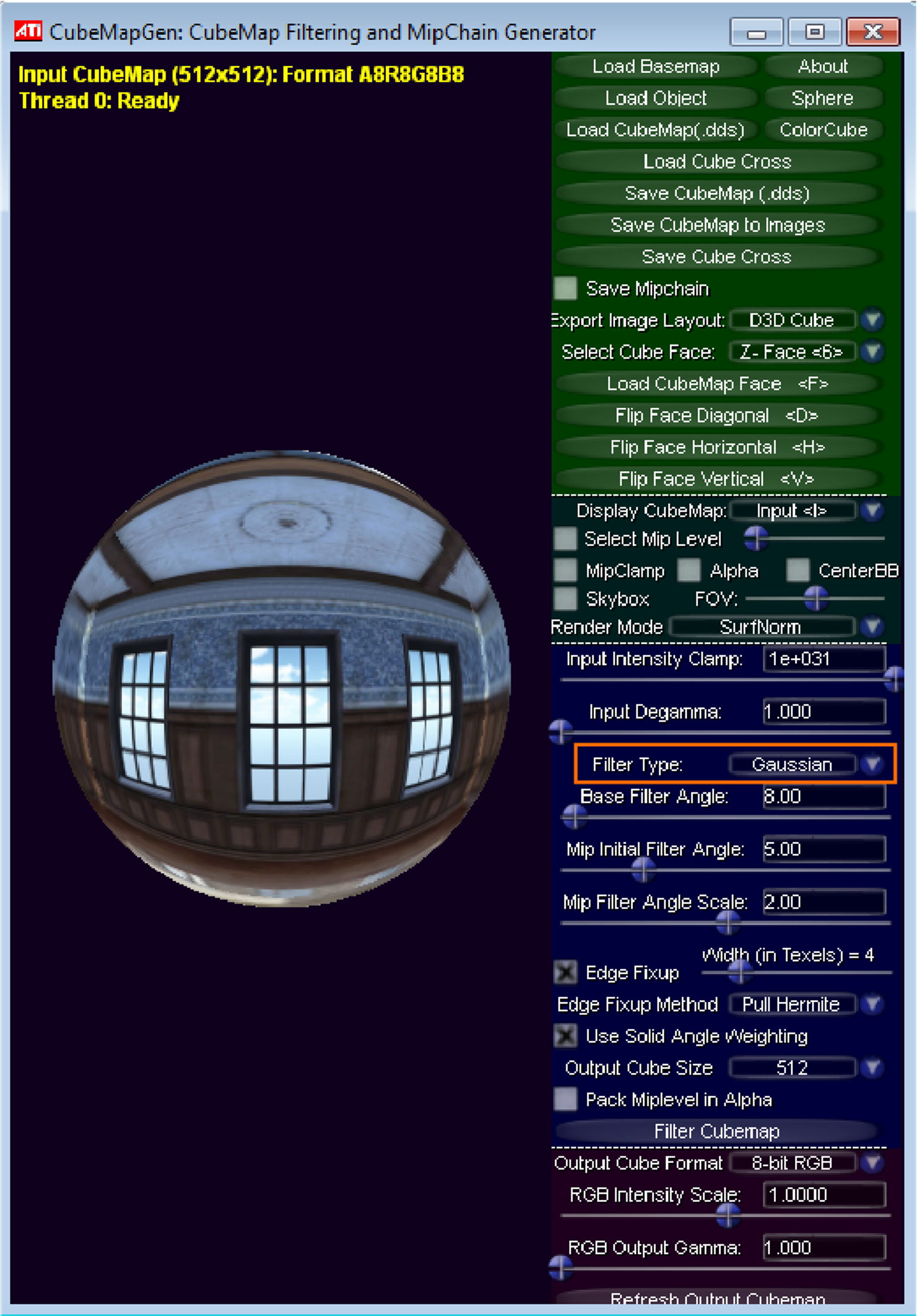The following screenshot shows the output result of CubeMapGen after applying a Gaussian filtering to achieve a frosty effect: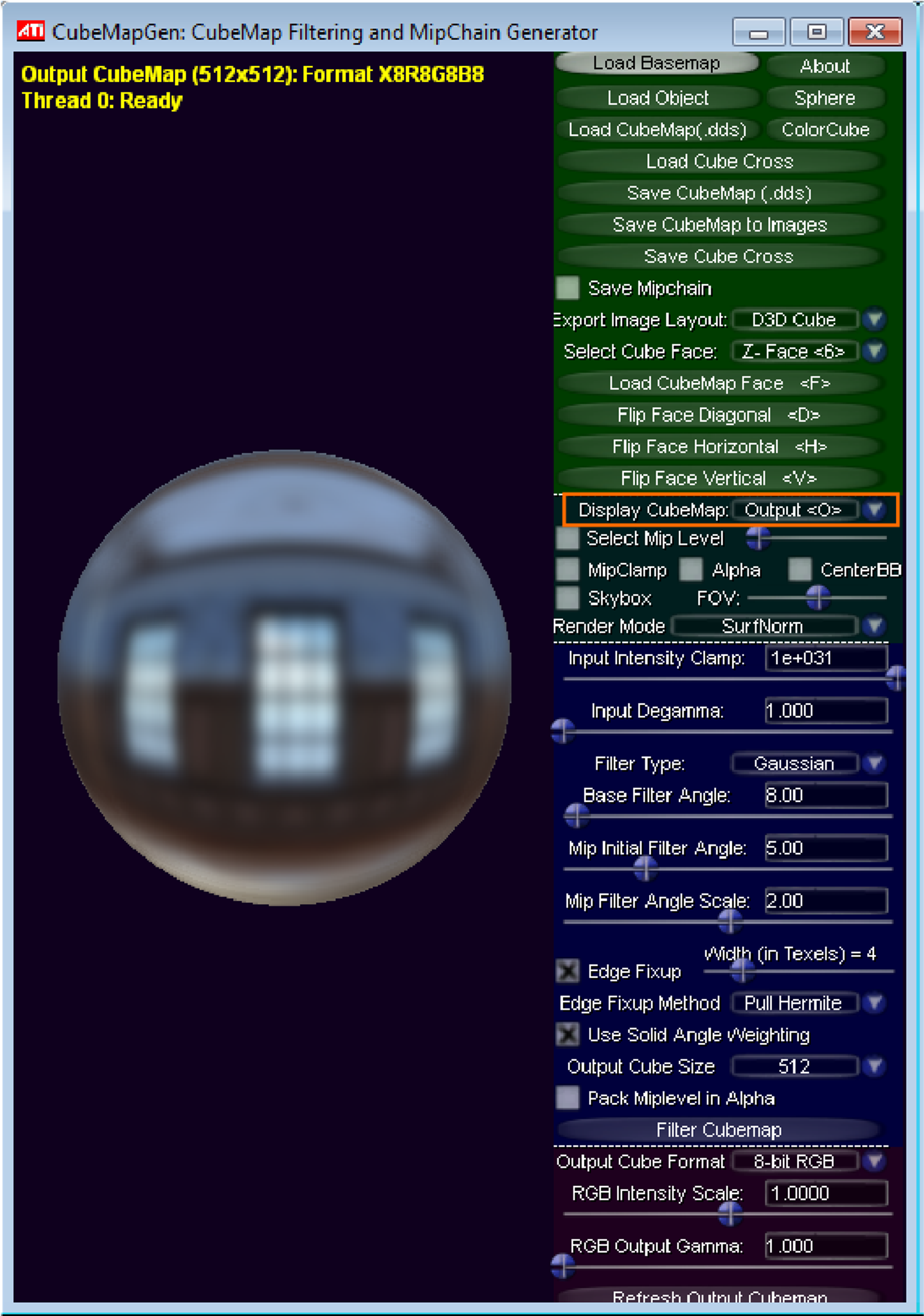The following table shows the filter parameters used with the Gaussian filter to achieve the frosty effect:

 Filter settings Value Type Gaussian Base Filter Angle 8 Mip Initial Filter Angle 5 Mip Filter Angle Scale 2.0 Edge Fixup Checked Edge Fixup Width 4

The following image shows a reflection that is generated with a cubemap with a frosty effect: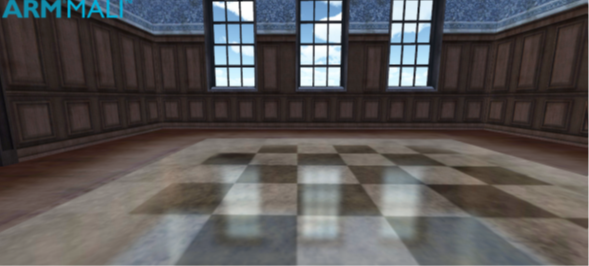The following image summarizes the preceding workflow that is used to apply filtering to Unity cubemaps with the CubeMapGen tool:#### Ray-box intersection algorithm

This section describes the ray-box intersection algorithm. The ray-box intersection algorithm was mentioned in Shader implementations.

Equation of a line:

`y = mx + b`

The vector form of this equation is:

`r = O + t*D`

Where

• `O` is the origin point
• `D` is the direction vector
• `t` is the parameter

The following image shows an axis aligned bounding box:The min and max points A and B define an axis-aligned bounding box `AABB`.

`AABB` defines a set of lines parallel to coordinate axis. The following equation defines each component of the line:

```x = Ax; y = Ay; z = Az
x = Bx; y = By; z = Bz
```

To find where a ray intersects one of those lines, make both equations equal. For example:

```Ox + tx*Dx = Ax
```

You can write the solution as:

`tAx = (Ax – Ox) / Dx`

Obtain the solution for all components of both intersection points in the same manner:

```tAx = (Ax – Ox) / Dx
tAy = (Ay – Oy) / Dy
tAz = (Az – Oz) / Dz
tBx = (Bx – Ox) / Dx
tBy = (By – Oy) / Dy
tBz = (Bz – Oz) / Dz
```

In vector form, they are:

```tA = (A – O) / D
tB = (B – O) / D
```

The solution finds where the line intersects the planes that are defined by the faces of the cube, but it does not guarantee that the intersections lie on the cube.

The following image shows a 2D representation of ray-box intersection:To find the solution that intersects with the box, you require the larger value of t for the intersection at the `min` plane:

```tmin = (tAx > tAy) ? tAx: tAy
tmin = (tmin > tAz) ? tmin: tAz
```

You require the smaller value of t for the intersection at the max plane:

```tmax = (tAx < tAy) ? tAx: tAy
tmax = (tmin < tAz) ? tmin: tAz
```

Note: The ray does not always intersect the box.

The following image shows a ray-box with no intersection:If you guarantee that the `BBox` encloses the reflective surface, that is, the origin of the reflected ray is inside the `BBox`. This means that there are always two intersections with the box, and the handling of different cases is simplified.

The following image shows a ray-box intersection in `BBox`:#### Source code for editor script to generate cubemaps

• This section provides the source code for the editor script to generate cubemaps:
```/*
* This confidential and proprietary software may be used only as
* authorised by a licensing agreement from Arm Limited* (C) COPYRIGHT 2014 Arm Limited* ALL
RIGHTS RESERVED
* The entire notice above must be reproduced on all authorised
* copies and copies may only be made to the extent permitted
* by a licensing agreement from Arm Limited.
*/
using UnityEngine;
using UnityEditor;
using System.IO;
/**
* This script must be placed in the Editor folder.
* The script renders the scene into a cubemap and optionally
* saves each cubemap image individually.
* The script is available in the Editor mode from the
* Game Object menu as "Bake Cubemap" option.
* Be sure the camera far plane is enough to render the scene.
*/
public class BakeStaticCubemap : ScriptableWizard
{
public Transform renderPosition;
public Cubemap cubemap;
// Camera settings.
public Color cameraBackgroundColor;
public float cameraNearPlane = 0.1f;
public float cameraFarPlane = 2500.0f;
public bool cameraUseOcclusion = true;
// Cubemap settings.
public FilterMode cubemapFilterMode = FilterMode.Trilinear;
// Quality settings.
public int antiAliasing = 4;
public bool createIndividualImages = false;
// The folder where individual cubemap images will be saved
static string imageDirectory = "Assets/CubemapImages";
static string[] cubemapImage = new string[]{"front+Z", "right+X", "back-Z", 	"left-X", "top+Y", "bottom-Y"};
static Vector3[] eulerAngles = new Vector3[]{new Vector3(0.0f,0.0f,0.0f), new 	Vector3(0.0f,-90.0f,0.0f), new Vector3(0.0f,180.0f,0.0f), new 	Vector3(0.0f,90.0f,0.0f), new Vector3(-90.0f,0.0f,0.0f), new 	Vector3(90.0f,0.0f,0.0f)};

void OnWizardUpdate()
{
helpString = "Set the position to render from and the cubemap to bake.";
if(renderPosition != null && cubemap != null)
{
isValid = true;
}
else
{
isValid = false;
}
}

void OnWizardCreate ()
{
// Create temporary camera for rendering.
GameObject go = new GameObject( "CubemapCam", typeof(Camera) );
// Camera settings.
go.camera.backgroundColor = cameraBackgroundColor;
go.camera.nearClipPlane = cameraNearPlane;
go.camera.farClipPlane = cameraFarPlane;
go.camera.useOcclusionCulling = cameraUseOcclusion;
// Cubemap settings
cubemap.filterMode = cubemapFilterMode;
// Set antialiasing
QualitySettings.antiAliasing = antiAliasing;
// Place the camera on the render position.
go.transform.position = renderPosition.position;
go.transform.rotation = Quaternion.identity;
// Bake the cubemap
go.camera.RenderToCubemap(cubemap);
// Rendering individual images
if(createIndividualImages)
{
if (!Directory.Exists(imageDirectory))
{
Directory.CreateDirectory(imageDirectory);
}
RenderIndividualCubemapImages(go);
}
// Destroy the camera after rendering.
DestroyImmediate(go);
}
void RenderIndividualCubemapImages(GameObject go)
{
go.camera.backgroundColor = Color.black;
go.camera.clearFlags = CameraClearFlags.Skybox;
go.camera.fieldOfView = 90;
go.camera.aspect = 1.0f;
go.transform.rotation = Quaternion.identity;
//Render individual images
for (int camOrientation = 0; camOrientation < eulerAngles.Length ; camOrientation++)
{
string imageName = Path.Combine(imageDirectory, cubemap.name + "_" + cubemapImage[camOrientation] + ".png");
go.camera.transform.eulerAngles = eulerAngles[camOrientation];
RenderTexture renderTex = new RenderTexture(cubemap.height, cubemap.height, cameraDepth);
go.camera.targetTexture = renderTex;
go.camera.Render();
RenderTexture.active = renderTex;
Texture2D img = new Texture2D(cubemap.height, cubemap.height, TextureFormat.RGB24, false);
img.ReadPixels(new Rect(0, 0, cubemap.height, cubemap.height), 0, 0);
RenderTexture.active = null;
GameObject.DestroyImmediate(renderTex);
byte[] imgBytes = img.EncodeToPNG();
File.WriteAllBytes(imageName, imgBytes);
AssetDatabase.ImportAsset(imageName, ImportAssetOptions.ForceUpdate);
}
AssetDatabase.Refresh();
}
static void RenderCubemap ()
{
ScriptableWizard.DisplayWizard("Bake CubeMap", typeof(BakeStaticCubemap),"Bake!");
}
}
```

## Combine reflections

This section shows you how to combine reflections. For example, reflections based on local cubemap techniques enable you to render high quality, efficient reflections based on static local cubemaps. However, if objects are dynamic, the static local cubemap is no longer valid and the technique does not work. You can solve this by combining static reflections with dynamically generated reflections. This is shown in the following image:If the reflective surface is planar, you can generate dynamic reflections with a mirrored camera. To create a mirrored camera, calculate the position and orientation of the main camera that renders the reflections at runtime. Mirror the position and orientation of the main camera, relative to the reflective plane.

The following image shows the mirrored camera technique:In the mirroring process, the new reflection camera ends up with its axis in the opposite orientation. In the same way that a physical mirror works, reflections from the left and right are inverted. Therefore, the reflection camera renders the geometry with an opposite winding.

To render the geometry correctly, you must invert the winding of the geometry before rendering the reflections. When you have finished rendering the reflections, restore the original winding.

The following steps image shows what is required to set up the mirrored camera and render the reflections:

1. Calculate reflection matrix `ReflMat` relative to reflection plane
2. Calculate the position of the reflection camera:
`reflCam.Pos= mainCam.Pos * ReflMat;`
3. Build world to camera matric for reflection camera:
`reflCam.WorldToCam = mainCam.WorldToCam * ReflMat;`
4. Set projection matrix for reflection camera:
`reflCam.ProjMat = mainCam.ProjMat;`
5. Set render texture:
```reflCam.SetRenderTex(reflTex);
reflMar.SetTex(_ReflTex, reflTex);```
6. Render reflections:
```GL.ReverseBackFacing(true);
reflCam.Render();
GL.ReverseBackFacing(false);```

Build the mirror reflection transformation matrix. Use this matrix to calculate the position, and the world-to-camera transformation matrix, of the reflection camera.

The following maths equation shows the mirror reflection transformation matrix:You can apply the reflection matrix transformation to the position and world-to-camera matrix of the main camera. This provides you with the position and world-to-camera matrix of the reflection camera.

The projection matrix of the reflection camera must be the same as the projection matrix of the main camera.

The reflection camera renders reflections to a texture.

For good results, you must set up this texture properly before rendering:

• Use mipmaps
• Set the filtering mode to trilinear
• Use multisampling

Ensure the texture size is proportional to the area of the reflective surface. The larger the texture is, the less pixelated the reflections are.

Note: Here is an example script for a mirrored camera.

This section shows you how to combine reflections in the shaders. You can combine static environment reflections with dynamic planar reflections in a shader. To combine reflections in the shaders, you must modify the shader code that we provided in Shader implementation.

The shader must incorporate the planar reflections that are rendered at runtime with the reflection camera. Therefore, the texture `_ReflectionTex` from the reflection camera, passes to the fragment shader as a uniform. The texture then combines with the planar reflection result using a `lerp()` function.

In addition to the data related to the local correction, the vertex shader also calculates the screen coordinates of the vertex using the built-in function `ComputeScreenPos()`. It passes these coordinates to the fragment shader, as you can see in the following code:

```vertexOutput vert(vertexInput input)
{
vertexOutput output;
output.tex = input.texcoord;
// Transform vertex coordinates from local to world.
float4 vertexWorld = mul(_Object2World, input.vertex);
// Transform normal to world coordinates.
float4 normalWorld = mul(float4(input.normal,0.0), _World2Object);
// Final vertex output position.
output.pos = mul(UNITY_MATRIX_MVP, input.vertex);
// ----------- Local correction ------------
output.vertexInWorld = vertexWorld.xyz;
output.viewDirInWorld = vertexWorld.xyz - _WorldSpaceCameraPos;
output.normalInWorld = normalWorld.xyz;
// ----------- Planar reflections ------------
output.vertexInScreenCoords = ComputeScreenPos(output.pos);
return output;
}
```

The planar reflections are rendered to a texture, so the fragment shader can access the screen coordinates of the fragment. To enable texture rendering, pass the vertex screen coordinates to the fragment shader as a varying.

• Apply the local correction to the reflection vector.
• Retrieve the color of the environment reflections `staticReflColor` from the local cubemap.

The following code shows how to combine static environment reflections. This code uses the local cubemap technique, with dynamic planar reflections, that are rendered at runtime using the mirrored camera technique:

```float4 frag(vertexOutput input) : COLOR
{
float4 staticReflColor = float4(1, 1, 1, 1);

// Find reflected vector in WS.
float3 viewDirWS = normalize(input.viewDirInWorld);
float3 normalWS = normalize(input.normalInWorld);
float3 reflDirWS = reflect(viewDirWS, normalWS);

// Working in World Coordinate System.
float3 localPosWS = input.vertexInWorld;
float3 intersectMaxPointPlanes = (_BBoxMax - localPosWS) / reflDirWS;
float3 intersectMinPointPlanes = (_BBoxMin - localPosWS) / reflDirWS;

// Look only for intersections in the forward direction of the ray.
float3 largestParams = max(intersectMaxPointPlanes, intersectMinPointPlanes);

// Smallest value of the ray parameters gives us the intersection.
float distToIntersect = min(min(largestParams.x, largestParams.y), largestParams.z);

// Find the position of the intersection point.
float3 intersectPositionWS = localPosWS + reflDirWS * distToIntersect;

// Get local corrected reflection vector.
float3 localCorrReflDirWS = intersectPositionWS - _EnviCubeMapPos;

// Lookup the environment reflection texture with the right vector.
float4 staticReflColor = texCUBE(_Cube, localCorrReflDirWS);

// Lookup the planar runtime texture
float4 dynReflColor = tex2Dproj(_ReflectionTex,
UNITY_PROJ_COORD(input.vertexInScreenCoords);

//Revert the blending with the background color of the reflection camera
dynReflColor.rgb /= (dynReflColor.a < 0.00392)?1:dynReflColor.a;

// Combine static environment reflections with dynamic planar reflections
float4 combinedRefl = lerp(staticReflColor.rgb, dynReflColor.rgb, dynReflColor.a);

// Lookup the texture color.
float4 texColor = tex2D(_MainTex, input.tex);
return _AmbientColor + texColor * _ReflAmount * combinedRefl;
}
```

The code performs the following operations:

• Extract the texture color `dynReflColor` from the planar run-time reflection texture `_ReflectionTex`.
• Declare `_ReflectionTex` as a uniform in the shader. If you also declare `_ReflectionTex` in the Property Block, you can see how it looks at runtime. This can assist you with debugging while you are developing your game.
• For the texture lookup, project the texture coordinates. You can use the Unity built-in function `UNITY_PROJ_COORD()`. This function divides the texture coordinates by the last component of the coordinate vector.
• Use the `lerp()` function to combine the static environment reflections and the dynamic planar reflections. For example, you could combine the following:
• The reflection color
• The texture color of the reflective surface
• The ambient color component

#### Combine reflections from a distant environment

This section shows you to combine reflections from a distant environment. When you render reflections from static and dynamic objects, you might also have to consider reflections from a distant environment. An example of a distant reflection is reflections from the sky that are visible through a window in your local environment.

Using the preceding example, you must combine three different types of reflections:

• Reflections from the static environment using the local cubemap technique.
• Planar reflections from dynamic objects using the mirrored camera technique.
• Reflections from the skybox using the standard cubemap technique. The reflection vector does not require a correction before fetching the texture from the cubemap.

For the skybox, we wish to ensure that it is only visible from the windows. To do this, render the transparency of the scene in the alpha channel when you are baking the static cubemap for reflections. Assign a value of one to opaque geometry and a value of zero where there is no geometry, or the geometry is fully transparent. For example, render the pixels that correspond to the windows with zero in the alpha channel.

Once we get to the shader code, we want to pass the skybox cubemap texture into the shaders as a uniform. We have called the skybox cubemap texture _Skybox in the code below.

To incorporate the reflections from a skybox, use the reflection vector `reflDirWS` to fetch the texel from the skybox cubemap.

Note: Do not apply a local correction.

In the fragment shader code that we show in Combine reflections shader implementation, find the following comment in the fragment shader code:

`// Lookup the planar runtime texture`

Insert the following lines immediately before the preceding comment:

```float4 skyboxReflColor = texCUBE(_Skybox, reflDirWS);
staticReflColor = lerp(skyboxReflColor.rgb, staticReflColor.rgb, staticReflColor.a);
```

This code combines the static reflections with reflections from the skybox.

The following image shows the result of combining different types of reflections: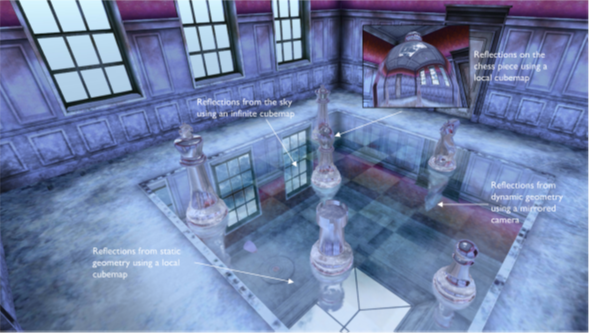## Dynamic soft shadows based on local cubemaps

Dynamic soft shadows based on local cubemaps uses a local cubemap to hold a texture that represents transparency of the static environment. This technique a very efficient technique that generates high-quality soft shadows.

In your scene, there are moving objects and static environments like rooms. By using this technique, you are not required to render static geometry to a shadow map every frame. This technique enables you to use a texture to represent the shadows.

Cubemaps can be a good approximation of a static local environment, including irregular shapes like the cave in the Ice Cave demo. The alpha channel can also represent the amount of light that enters the room.

The objects that move are typically everything except the room, for example:

• The sun
• The camera
• Dynamic objects

When the whole room is represented by a cube texture, you can access arbitrary texels of the environment within a fragment shader. For example, the sun can be in any arbitrary position and you can calculate the amount of light reaching a fragment based on the value fetched from the cubemap.

The alpha channel, or transparency, represents the amount of light that enters the local environment. In your scene, pass the cubemap texture through to the fragment shaders that render the static and dynamic objects that want shadows added.

The following instructions describe how to generate shadow cubemaps:

1. Start with a local environment that you want to add shadows to, from light sources outside of the environment. For example, a room, a cave, or a cage.
2. This technique is like reflections based on a local cubemap. For more information, see Implement reflections with a local cubemap.
3. Create the shadow cubemap in the same way that you created the reflection cubemap, then add an alpha channel. This channel represents the amount of light that enters the local environment.
4. Calculate the position to render the six faces of the cubemap from. Usually the render position is in the center of the bounding box of the local environment. This position is required for the generation of the cubemap. The position also must be passed to the shaders to calculate a local correction vector to fetch the right texel from the cubemap.
5. When you have decided where to position the center of the cubemap you can render all the faces to the cubemap texture. This means that you can record the transparency, or alpha, of the local environment. The more transparent an area is, the more light comes into the environment. If there is no geometry, the texture is fully transparent. If necessary, you can use the RGB channels to store the color of the environment for colored shadows, for example stained glass, reflections, or refractions.

The following instructions briefly describe how to render shadows:

1. Build a vector `PiL` in world space from a vertex or fragment, to the light or lights, and fetch the cubemap shadow using this vector.
2. Before fetching each texel, you must apply local correction to the `PiL` vector.
Note: Arm recommends you make the local correction in the fragment shader to obtain more accurate shadows.
3. To compute the local correction, you must calculate an intersection point of the fragment-to-light vector with the bounding box of the environment. Use this intersection point to build another vector from the cubemap origin position `C` to the intersection point `P`. This computes the output value `CP`, which is the corrected fragment-to-light vector that you use to fetch a texel from the shadow cubemap.

The following input parameters are required to calculate the local correction:

`_EnviCubeMapPos`
The cubemap origin position
`_BBoxMax`
The maximum point of the bounding box of the environment
`_BBoxMin`
The minimum point of the bounding box of the environment
`Pi`
The fragment position in world space
`PiL`
The normalized fragment-to-light vector in world space

The following image shows the local correction of the fragment-to-light vector:The following example code shows how to calculate the correct `CP` vector:

```// Working in World Coordinate System.
vec3 intersectMaxPointPlanes = (_BBoxMax - Pi) / PiL;
vec3 intersectMinPointPlanes = (_BBoxMin - Pi) / PiL;
// Looking only for intersections in the forward direction of the ray.
vec3 largestRayParams = max(intersectMaxPointPlanes, intersectMinPointPlanes);
// Smallest value of the ray parameters gives us the intersection.
float dist = min(min(largestRayParams.x, largestRayParams.y), largestRayParams.z);
// Find the position of the intersection point.
vec3 intersectPositionWS = Pi + PiL * dist;
// Get the local corrected vector.
CP = intersectPositionWS - _EnviCubeMapPos;
```

Use the `CP` vector to fetch a texel from the cubemap. The alpha channel of the texel provides information about how much light or shadow you must apply to a fragment:

`float shadow = texCUBE(cubemap, CP).a;`

The following image shows a chess room with hard shadows: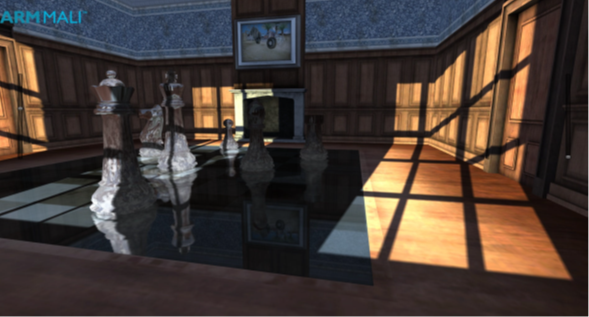This technique generates working shadows in your scene, but you can improve the quality of the shadows with two more steps:

• Smoothness
The cubemap shadow technique does not use depth information to apply shadows. Therefore, some faces are incorrectly lit when they are meant to be in shadow.
The problem only occurs when a surface is facing in the opposite direction to the light. To fix this problem, check the angle between the normal vector and the fragment-to-light vector, `PiL`. If the angle, in degrees, is outside of the range -90 to 90, the surface is in shadow.
The following code snippet checks to see if it outside the range:
`if (dot(PiL,N) < 0) shadow = 0.0;`
The preceding code causes a hard switch from light to shade. For a smoother transition, use the following formula:
`shadow *= max(dot(PiL, N), 0.0);`
Here is what the formula does:
• `shadow` is the alpha value fetched from the shadow cubemap
• `PiL` is the normalized fragment-to-light vector in world space
• `N` is the normal vector of the surface in world space
The following image shows a chess room with back faces in shadow: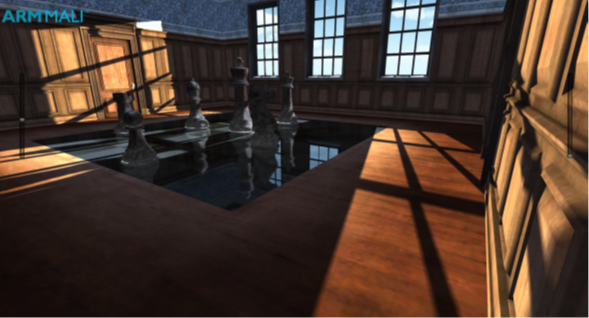Smoothness
This shadow technique can provide realistic soft shadows in your scene. The following steps explain how you can apply smoothness:
1. Generate mipmaps and set trilinear filtering for the cubemap texture.
2. Measure the length of a fragment-to-intersection-point vector.
3. Multiply the length by a coefficient.
The coefficient is a normalizer of a maximum distance in your environment to the number of mipmap levels. You can calculate the coefficient automatically against bounding volume and mipmap levels. You must customize the coefficient to your scene, enabling you to tweak the settings to suit the environment, improving visual quality. For example, the coefficient used in the Ice Cave project is 0.08.
You can reuse the results from the calculations that you did for the local correction. This is shown in the following steps:
1. Create a variable `texLod` for the length of the segment from the fragment position to the intersection point of the fragment-to-light vector with the bounding box. Set this variable to `dist` from the code snippet for local correction.
2. This is shown in the following code:
`float texLod = dist;`
3. Multiply `texLod` by the distance coefficient:
`texLod *= distanceCoefficient;`
4. To implement softness, fetch the correct mipmap level of the texture using the Cg function `texCUBElod()` or the GLSL function `textureLod()`.
5. Construct a `vec4` where `XYZ` represents a direction vector and the `W` component represents the LOD, as shown in the following code:
```CP.w = texLod;

The following image shows a chess room with smooth shadows: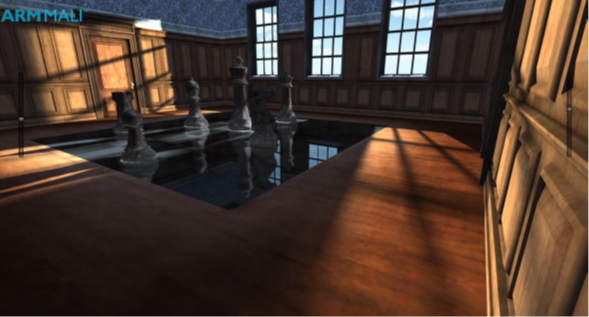To get shadows that are complete with dynamic content, you must combine cubemap shadows with a traditional shadow map technique. Combing cubemap shadows with a traditional shadow map technique is more work, but it is worth the extra work because you are only required to render dynamic objects to the shadow map. This is shown when comparing the following two images:

The following image shows the chess room with smooth shadows only: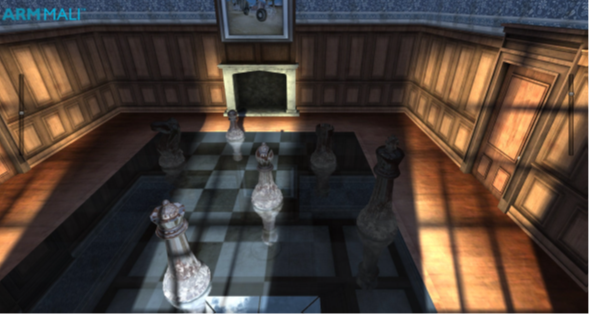The following image shows the chess room with smooth shadows combined with dynamic shadows: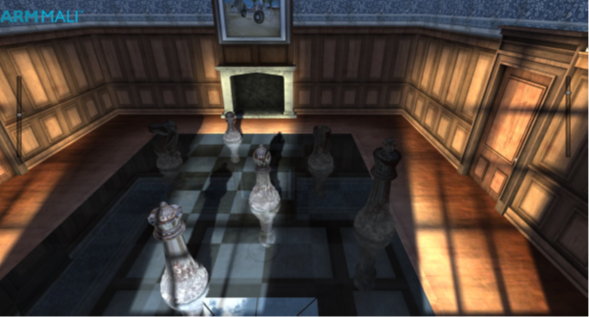#### Results of the cubemap shadow technique

This section shows you the results of the cubemap shadow technique, which was introduced to you in Combine cubemap shadows with a shadow map.

In traditional techniques, rendering shadows can be quite expensive because it involves rendering the whole scene from the perspective of each shadow-casting light source. The cubemap shadow technique described here delivers improved performance because it is mostly pre-baked.

The cubemap shadow technique is also independent of output-resolution. It produces the same visual quality at 1080p, 720p, and other resolutions.

The softness filtration is calculated in hardware, so the smoothness comes at almost no computational cost. The smoother the shadow, the more efficient the technique is. This is because the smaller mipmap levels result in less data than traditional shadow map techniques. Traditional techniques require a large kernel to make shadows smooth enough to be more visually appealing. Therefore, this requires high memory bandwidth, therefore, reducing performance.

The quality that you get with the cubemap shadow technique is higher than you might expect. It provides realistic softness and stable shadows with no shimmering at the edges. Shimmering edges can be observed when using traditional shadow map techniques because of rasterization and aliasing effects. However, none of the anti-aliasing algorithms can fix this problem entirely.

The cubemap shadow technique does not have a shimmering problem. The edges are stable even if you are using a much lower resolution than the one that is used in the render target. You can use four times lower resolution than the output and there are not artifacts or unwanted shimmering. Using four times lower resolution also saves memory bandwidth, therefore, improving performance.

Note: This technique can be used on any device on the market that supports shaders like OpenGL ES 2.0 or higher. It combines well with the reflections that are based on local cubemaps technique, making shadows an easy addition.

Note: The technique cannot be used for everything in your scene. Dynamic objects, for instance, receive shadows from the cubemap but they cannot be pre-baked to the cubemap texture. For dynamic objects, use shadow maps for generating shadows, blended with the cubemap shadow technique.

The following image shows the Ice Cave with shadows: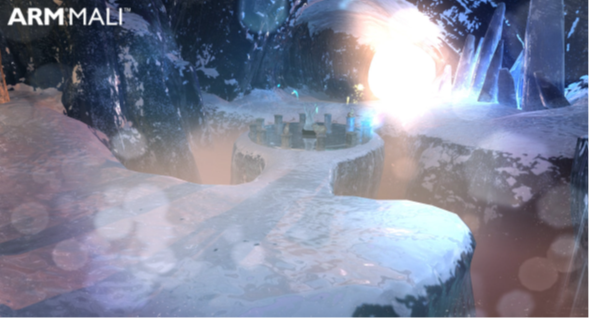The following images show the Ice Cave with smooth shadows: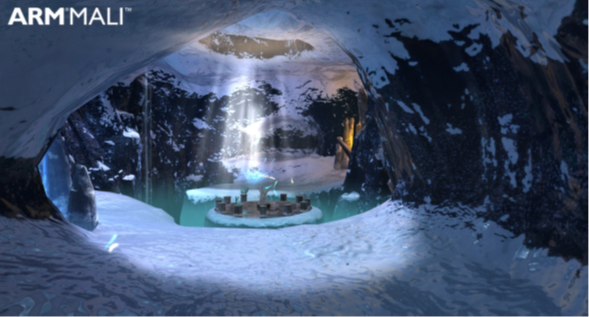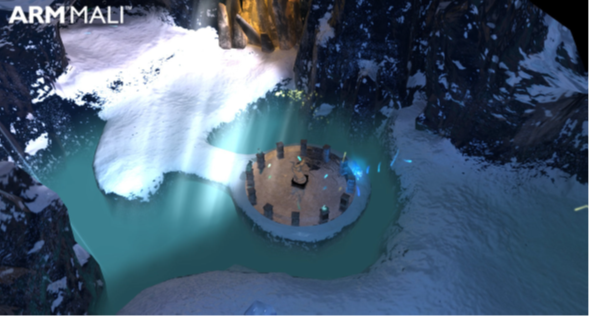## Refractions based on local cubemaps

You can use local cubemaps to implement high-quality refractions. Refraction is defined as the bending of light as it passes from one medium, with refractive index n1 to another medium with refractive index n2.You can combine the refractions calcualted from local cubemaps  with reflections at runtime.

Game developers are regularly looking for efficient methods to implement visually impressive effects in their games, especially when targeting mobile platforms. This is because you must carefully balance resources to achieve maximum performance.

Refraction is the change in direction of a light wave because of a change in the medium that the light wave is passing through. If you want extra realism with semi-transparent geometry, refraction is an important effect to consider.

The refractive index determines how much light is bent, or refracted, when entering a material.

You use Snell’s Law to calculate the relationship between the refractive indices and the sine of the incident and refracted angles.

The following image shows the relationship between Snell’s Law and refraction:#### Refraction implementatons

This section describes some refraction implementations. Developers have tried to render refraction since they started to render reflections. This is because these processes take place together in any semi-transparent surface. There are several techniques for rendering reflections, but not many for refraction.

Existing methods for implementing refraction at runtime differ depending on the specific type of refraction. Most of the techniques render the scene behind the refractive object to a texture at runtime. To achieve the refracted appearance, apply a texture distortion in a second pass. Depending on the texture distortion, you can use this approach to render refraction effects like water, heat haze, glass, and other effects.

Some of these techniques can achieve good results. But the texture distortion is not physically based. This means that the results are not always correct. For example, if you render a texture from the point of view of the refraction camera, there might be areas that are not directly visible to the camera. But these areas are visible in a physically based refraction.

The main limitation of using render-to-texture methods is quality. When the camera is moving, pixel shimmering or pixel instability is often visible.

#### About static cubemaps to implement reflections or refractions

Local cubemaps are an excellent technique for rendering reflections. Developers have used static cubemaps to implement both reflections and refractions.

However, if you use static cubemaps to implement reflections or refractions in a local environment, the results are incorrect if you do not apply a local correction. Therefore, a local correction is applied to ensure correct results. Local correction is a technique that is highly optimized. It is especially useful for mobile devices where run-time resources are limited and must be carefully balanced.

#### Prepare the cubemap

You must prepare the cubemap to be used in the refraction implementation.

To prepare the cubemap, follow these steps:

1. Place a camera in the center of the refractive geometry.
2. Hide the refractive object and render the surrounding static environment to a cubemap in the six directions. You can use this cubemap for implementing both refraction and reflection.
3. Bake the environment surrounding the refractive object into a static cubemap.
4. Determine the direction of the refraction vector and find where it intersects with the bounding box of the local environment.
5. Apply the local correction in the same way that is shown in Dynamic soft shadows based on local cubemaps.
6. Build a new vector from the position where the cubemap was generated, to the intersection point. Use this final vector to fetch the texel from the cubemap, to render what is behind the refractive object.

Instead of fetching the texel from the cubemap using the refracted vector `Rrf`, you find the point `P` where the refracted vector intersects the bounding box. Then build a new vector `R'rf` from the center of the cubemap `C` to the intersection point `P`. Use this new vector to fetch the texture color from the cubemap where the refracted vector intersects the bounding box. This is shown in the following code:

```float eta=n2/n1;
float3 Rrf = refract(D,N,eta);
```

Find intersection point `P`

Find vector `R'rf = CP;`

`float4 col = texCube(Cubemap, R'rf);`

The following image shows a scene with a cubemap and the refraction vectors:The refraction that is produced by this technique is realistic, because it uses real-world physics.  The direction of the refraction vector is calculated using Snell’s Law. The implementation that is shown in the preceding image uses a built-in function to find the refraction vector `R` strictly according to Snell's law:

`R = refract( I, N, eta);`

Where:

• `I` is the normalized view or incident vector
• `N` is the normalized normal vector
• `eta` is the ratio of indices of refractions n1/n2

The following image shows the flow of shaders that implement refraction based on a local cubemap:This section shows you to use shader implementation.

When you fetch the texel corresponding to the locally corrected refraction direction, you might want to combine the refraction color with other lighting. For example, reflections that take place simultaneously with refraction.

To combine the refraction color with other lighting, you must pass an extra view vector to the fragment shader and apply the local correction to it. Use the result to fetch the reflection color from the same cubemap.

The following code shows how to combine reflection and refraction to produce the final output color:

```// ----------------- Environment reflections ----------------
float3 newReflDirWS = LocalCorrect(input.reflDirWS, _BBoxMin, _BBoxMax, input.posWorld, _EnviCubeMapPos);
float4 staticReflColor = texCUBE(_EnviCubeMap, newReflDirWS);
// ----------------- Environment refractions ----------------
float3 newRefractDirWS = LocalCorrect(RefractDirWS, _BBoxMin, _BBoxMax, input.posWorld, _EnviCubeMapPos);
float4 staticRefractColor = texCUBE(_EnviCubeMap, newRefractDirWS);
// ----------------- Combined reflections and refractions ----------------
float4 combinedReflRefract = lerp(staticReflColor, staticRefractColor, _ReflAmount);
float4 finalColor = _AmbientColor + combinedReflRefract;
```

The coefficient `_ReflAmount` is passed as a uniform to the fragment shader. Use this coefficient to adjust the balance between reflection and refraction contributions. You can manually adjust `_ReflAmount` to achieve the visual effect you require.

Note: You can find the implementation of the LocalCorrect function in our blog Reflections based on local cubemaps in Unity.

When the refractive geometry is a hollow object, refractions and reflections take place in both the front and back surfaces.

The following image shows refraction on a glass bishop based on a local cubemap: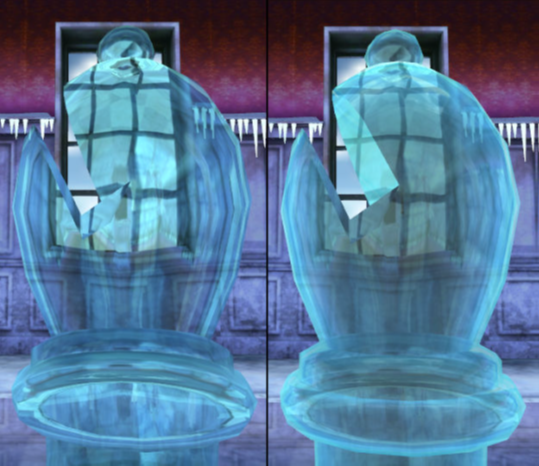The glass bishop on the left shows the first pass that renders only back faces with local refraction and reflections.

The glass bishop on the right shows the second pass renders only front faces with local refraction and reflections, and alpha blending with the first pass.

This process performs the following steps:

• In the first pass, render the semi-transparent object in the same manner that you render opaque geometry. Render the object last with front-face culling on. That is, only render the back faces. You do not want to occlude other objects, so do not write to the depth buffer.
• The color of the back face is obtained by mixing the colors that are calculated from the reflection, refraction, and the diffuse color of the object itself.
• In the second pass, render the front faces with back face culling. This operation must be done last in the rendering queue. Ensure depth writing is off. Obtain the front-face color by mixing the refraction and reflection textures with the diffuse color. The refraction in the second pass adds more realism to the final rendering. You can skip this step if the refraction on the back faces is enough to highlight the effect.
• In the final pass, alpha blend the resulting color with the first pass.

The following image shows the result of implementing refractions based on a local cubemap, on a semitransparent phoenix in the Ice Cave demo: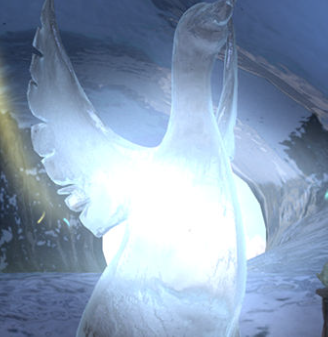The following image shows a semi-transparent phoenix wing: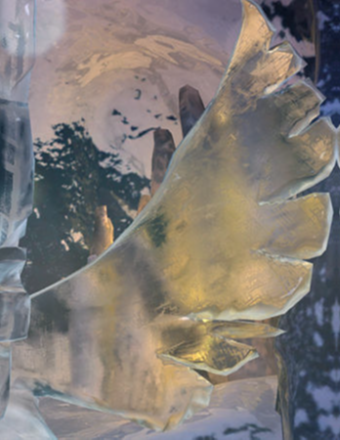## Next steps

This guide has introduced you to some rendering techniques using local cubemaps. We have looked at implementing reflections with a local cubemap, combining reflections, and refractions based on a local cubemap.

After reading this guide, you will be ready to implement some of the techniques into your own Unity programs. To keep learning about advanced graphics in Unity, read the next guide in our series: Special effects graphic techniques.